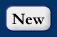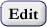## DataTypeCV Values

Used to populate the DataType field of the Variables tableTerm DefinitionAverage The values represent the average over a time interval, such as daily mean discharge or daily mean temperature.Best Easy Systematic Estimator Best Easy Systematic Estimator BES = (Q1 +2Q2 +Q3)/4. Q1, Q2, and Q3 are first, second, and third quartiles. See Woodcock, F. and Engel, C., 2005: Operational Consensus Forecasts.Weather and Forecasting, 20, 101-111. (http://www.bom.gov.au/nmoc/bulletins/60/article_by_Woodcock_in_Weather_and_Forecasting.pdf) and Wonnacott, T. H., and R. J. Wonnacott, 1972: Introductory Statistics. Wiley, 510 pp.Categorical The values are categorical rather than continuous valued quantities. Mapping from Value values to categories is through the CategoryDefinitions table.Constant Over Interval The values are quantities that can be interpreted as constant for all time, or over the time interval to a subsequent measurement of the same variable at the same site.Continuous A quantity specified at a particular instant in time measured with sufficient frequency (small spacing) to be interpreted as a continuous record of the phenomenon.Cumulative The values represent the cumulative value of a variable measured or calculated up to a given instant of time, such as cumulative volume of flow or cumulative precipitation.Incremental The values represent the incremental value of a variable over a time interval, such as the incremental volume of flow or incremental precipitation.Maximum The values are the maximum values occurring at some time during a time interval, such as annual maximum discharge or a daily maximum air temperature.Median The values represent the median over a time interval, such as daily median discharge or daily median temperature.Minimum The values are the minimum values occurring at some time during a time interval, such as 7-day low flow for a year or the daily minimum temperature.Mode The values are the most frequent values occurring at some time during a time interval, such as annual most frequent wind direction.Regular Sampling Scheduled sampling at regular time increments with a frequency that is too coarse for interpreting records as continuous but with a resolution that is representative of the time scale of fluctuation of the phenomenon.Sporadic The phenomenon is sampled at a particular instant in time but with a frequency that is too coarse for interpreting the record as continuous. This would be the case when the spacing is significantly larger than the support and the time scale of fluctuation of the phenomenon, such as for example infrequent water quality samples.StandardDeviation The values represent the standard deviation of a set of observations made over a time interval. Standard deviation computed using the unbiased formula SQRT(SUM((Xi-mean)^2)/(n-1)) are preferred. The specific formula used to compute variance can be noted in the methods description.Unknown The data type is unknownVariance The values represent the variance of a set of observations made over a time interval. Variance computed using the unbiased formula SUM((Xi-mean)^2)/(n-1) are preferred. The specific formula used to compute variance can be noted in the methods description.